# [软件][编程][研究] CASD: Calculate Average and Standard Deviation Curves [平均与标准偏差曲线计算工具]

• 程序图标 ( Icon )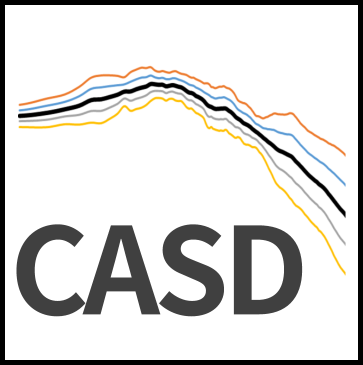• 程序介绍 ( Introduction)

The basic function of the program is to calculate the average and standard deviation curves. The program can consider Normal distribution and Log-normal distribution models for evaluation, and can save the analysis results as a text file or directly output Excel charts.

• 程序使用 ( Examples )

STEP 1：准备曲线数据，将曲线数据按以下格式准备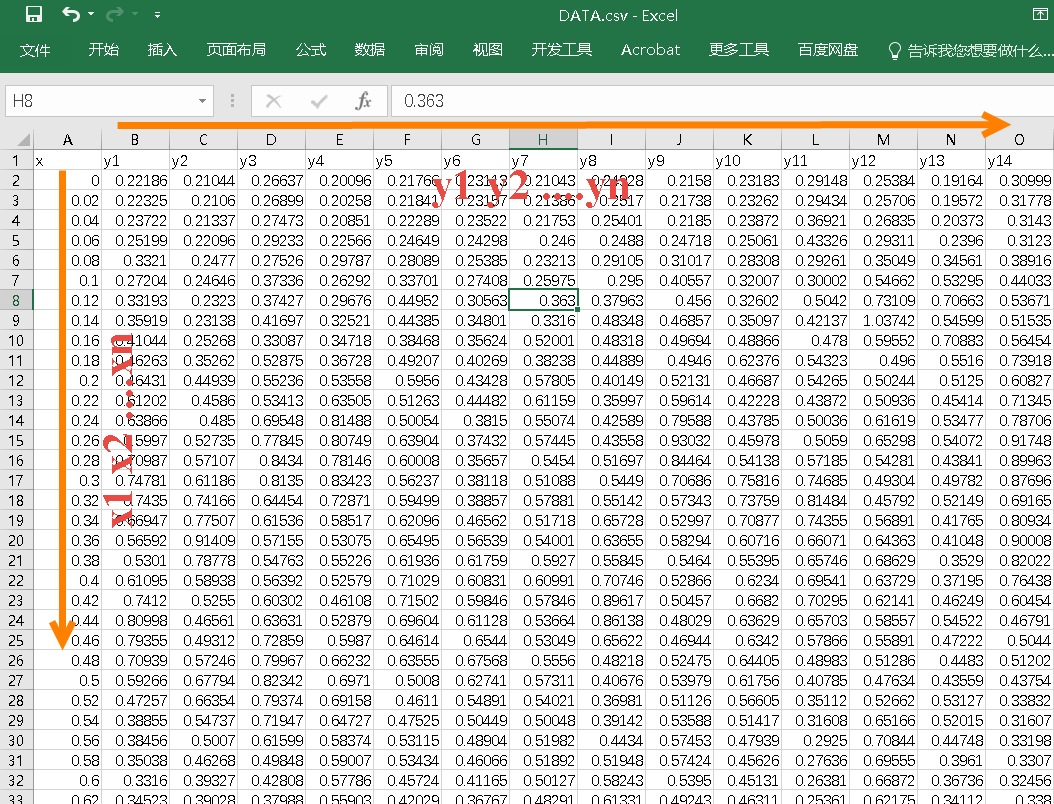STEP 2：打开软件，导入数据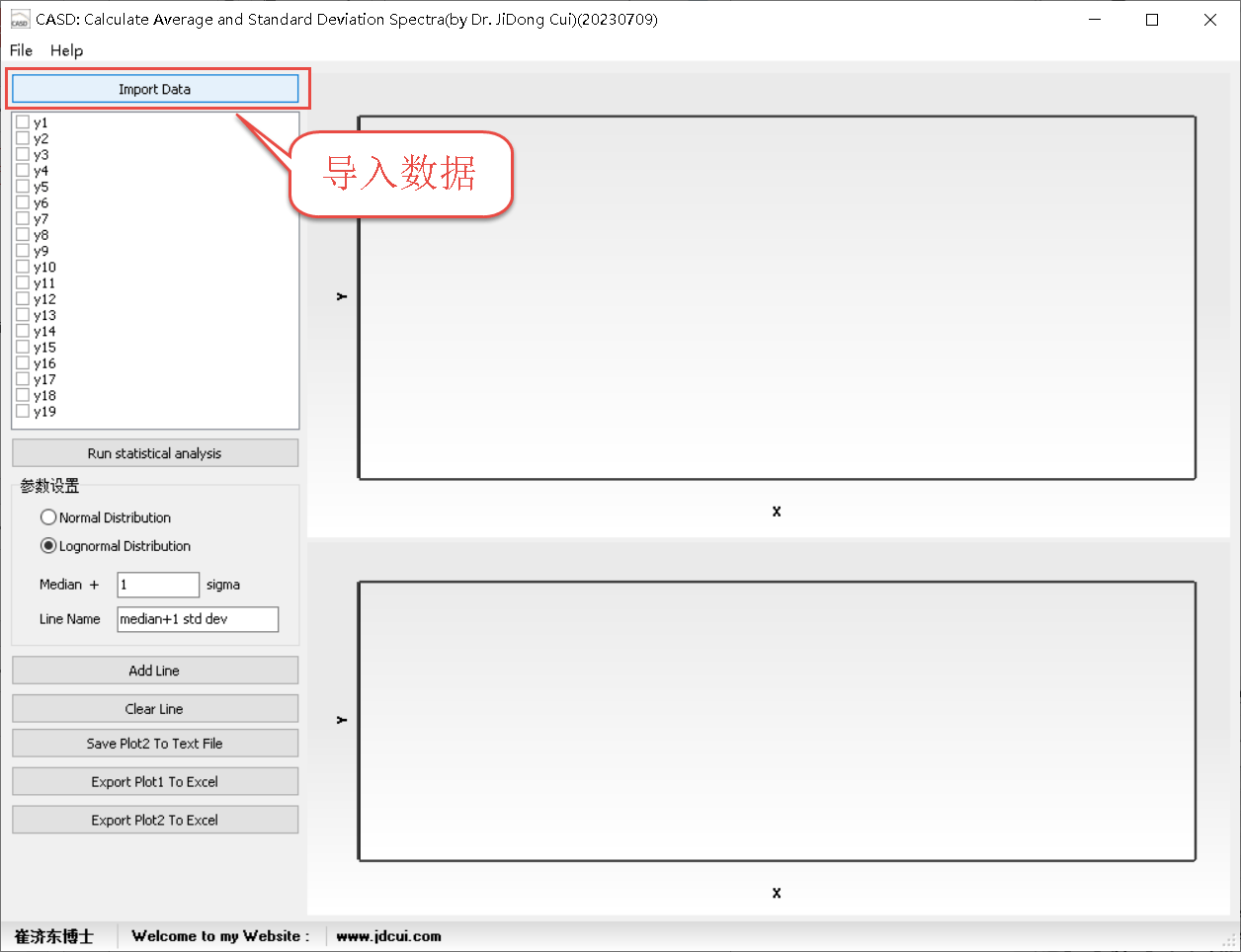STEP 3:  对需要分析的数据进行打勾，并点击Run statistical analyis 进行分析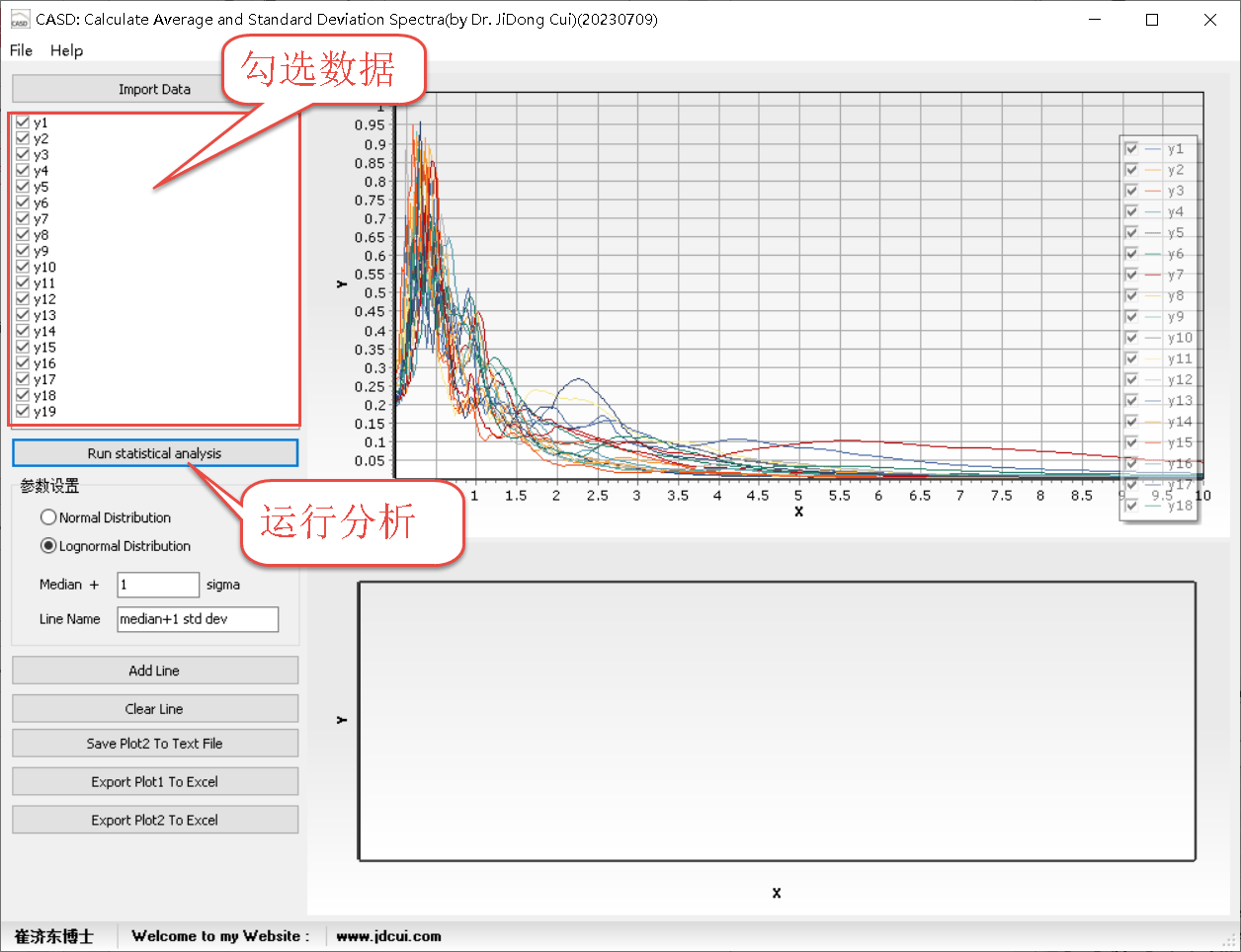STEP 4: 选择对数正态分布或者正态分布，并指定偏差倍数及曲线名称，点击 Add Line 进行曲线添加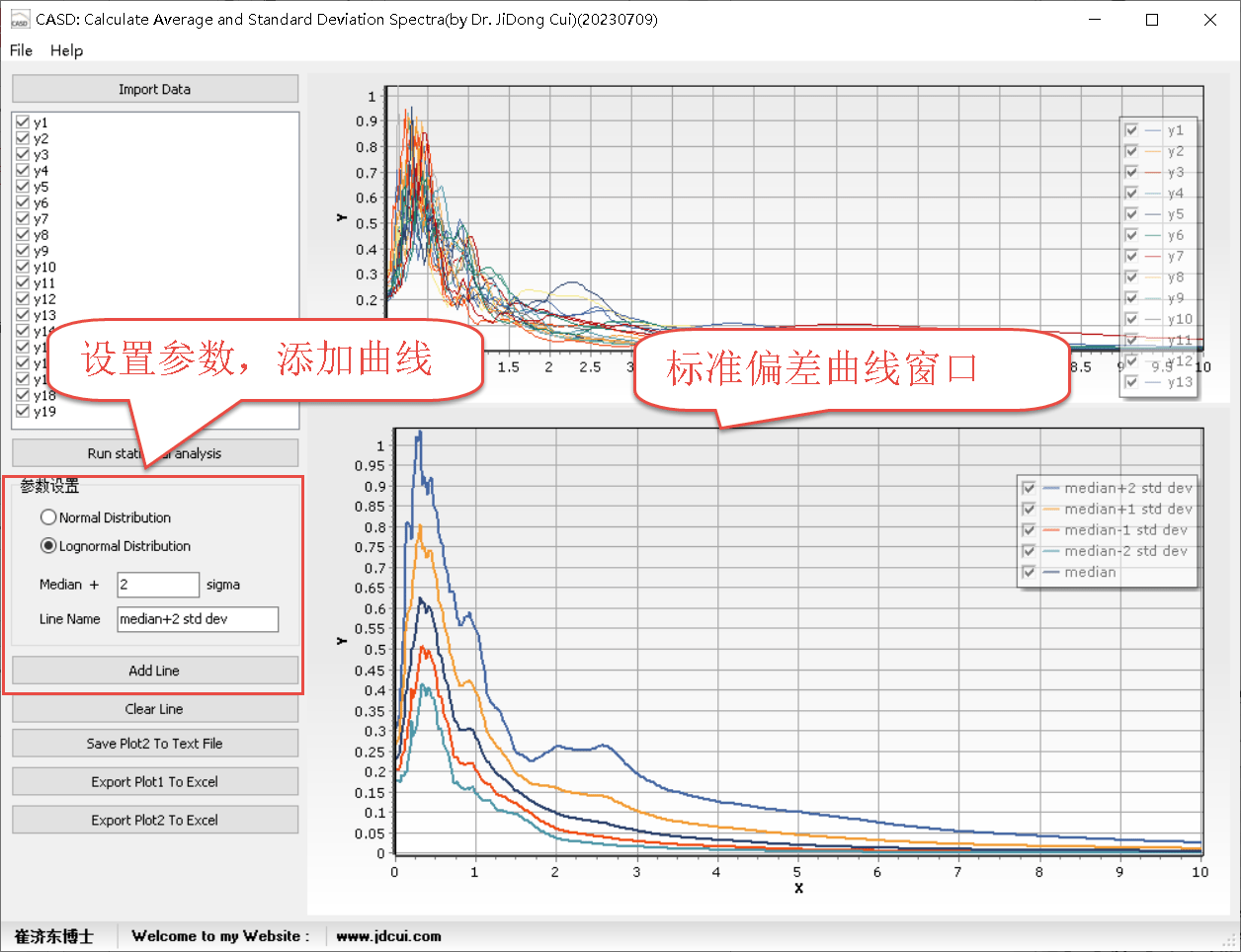STEP 5:  保存文本或者输出EXCEL图表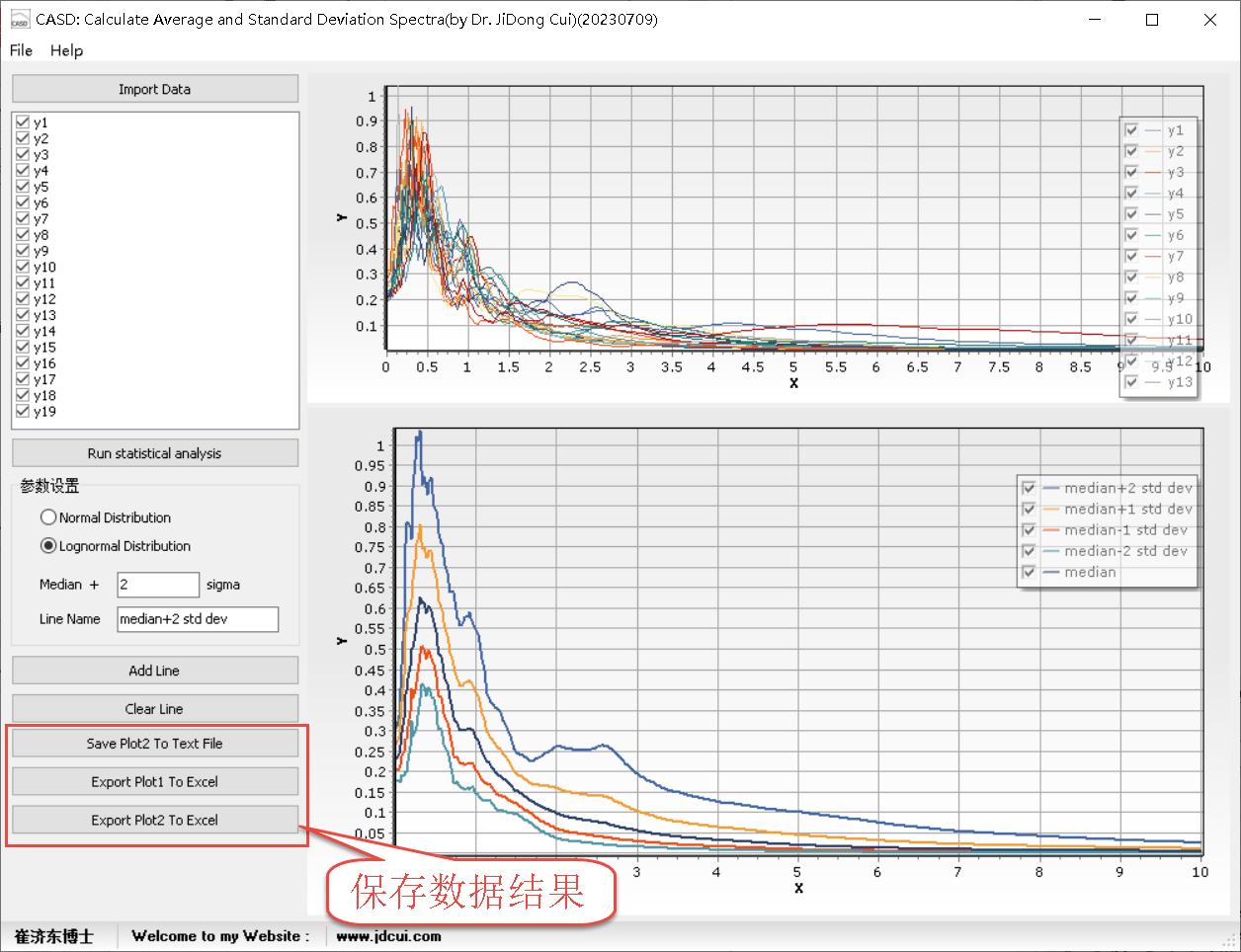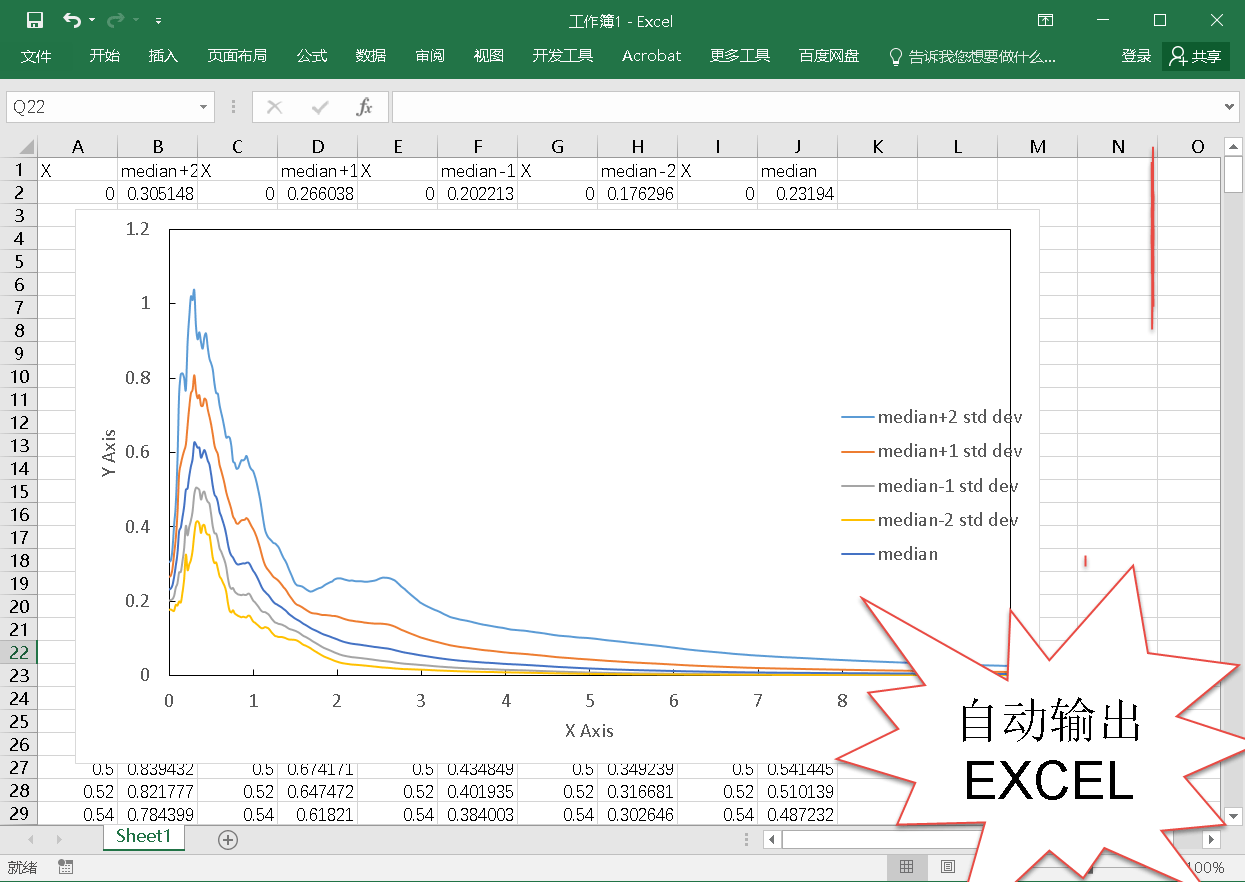STEP 6:  根据需要，自行对图表进行处理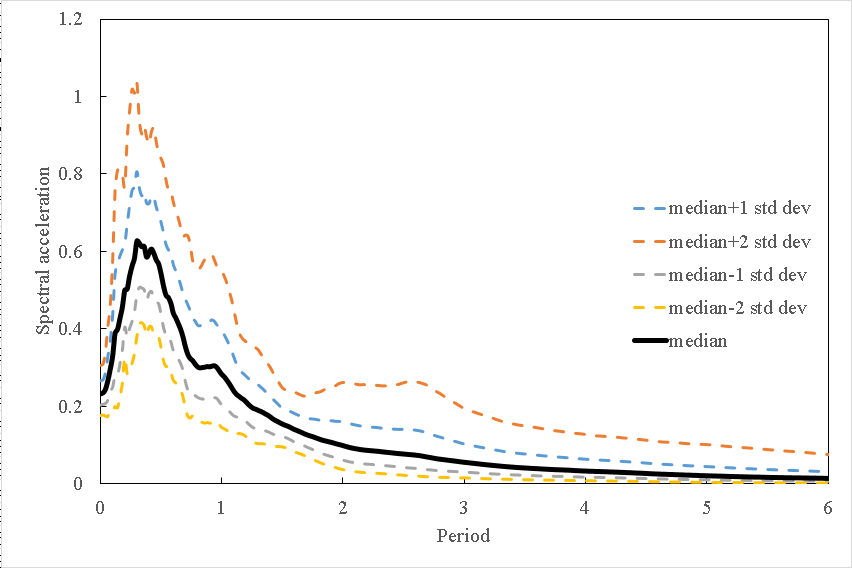• 微信公众号 ( Wechat Subscription)### 1 thought on “[软件][编程][研究] CASD: Calculate Average and Standard Deviation Curves [平均与标准偏差曲线计算工具]”

1.